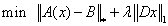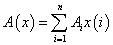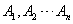# Mixed norm minimization

how to solve this problem using CVX :for some positive lambda , where the norm of the first argument is the nuclear norm
and the mapping A is defined as :the mapping is an affine mapping ofcourse and i can construct using a matlab code , and the matrix B has same dimension as A(x), i don’t know how to represent a general mapping from vector to matrix in CVX and for the first norm term we can separate it from the objective and make an SDP constraint

You can use `norm_nuc` for the nuclear norm.

As for the mysterious unspecified linear map `A(x)`, my some advice/question from How to write the following in CVX? applies. Show us the MATLAB code to evaluate `A(x)` when `x` is a numerically-populated MATLAB vector.

it can be written as :Show the MATLAB code, not some vague mathematical formula.

i haven’t been able to write the code yet(for the whole optimization problem) , my question is clear , imagine u have such mapping with known mapping matricesand the mapping is defined as above from vector x to some matrix A(x) , how to code this in cvx , ofcourse i constructed the mapping in matlab but its a trivial one ,u give it x and it gives u the resulting matrix , i think i made my self clear, or how can i call mapping function inside the cvx if this maybe possible

what about the l1 norm , imagine that i have the mapping as a script function called func(x), can u write the cvx version of the whole problem

Assume `A` is p by n by q and `A(:,:,i)` is A_i for i from 1 to q.
is this what you want?

``````cvx_begin
variable x(n)
linear_map = 0
for i = 1:q
linear_map = linear_map + A(:,:,i)*x(i)
end
minimize(norm_nuc(linear_map - B) + lambda*norm(D*x,1))
cvx_end``````

This should give you the idea. If that is not the correct linear map, then change it to what is. The changes to the MATLAB version and the CVX version should be the same, presuming you don’t use implicit arithmetic expansion https://blogs.mathworks.com/loren/2016/10/24/matlab-arithmetic-expands-in-r2016b/ which was introduced in MATLAB R2016b but is not supported in CVX.